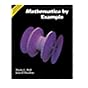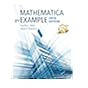Normal view

# Mathematica by example [electronic resource] / Martha L. Abell and James P. Braselton.

Material type:TextPublisher: Amsterdam ; Boston : Elsevier Academic Press, �2004Edition: 3rd edDescription: 1 online resource (xii, 571 pages) : illustrationsContent type: text Media type: computer Carrier type: online resourceISBN: 9780080491790; 0080491790Genre/Form: Electronic books.Additional physical formats: Print version:: Mathematica by example.DDC classification: 510/.285536 LOC classification: QA76.95 | .A214 2004ebOnline resources: Click here to access online
Contents:
Cover -- Copyright Page -- Contents -- Preface -- Chapter 1. Getting Started -- 1.1 Introduction to Mathematica -- 1.2 Loading Packages -- 1.3 Getting Help from Mathematica -- Chapter 2. Basic Operations on Numbers, Expressions, and Functions -- 2.1 Numerical Calculations and Built-In Functions -- 2.2 Expressions and Functions: Elementary Algebra -- 2.3 Graphing Functions, Expressions, and Equations -- 2.4 Solving Equations -- Chapter 3. Calculus -- 3.1 Limits -- 3.2 Differential Calculus -- 3.3 Integral Calculus -- 3.4 Series -- 3.5 Multi-Variable Calculus -- Chapter 4. Introduction to Lists and Tables -- 4.1 Lists and List Operations -- 4.2 Manipulating Lists: More on Part and Map -- 4.3 Mathematics of Finance -- 4.4 Other Applications -- Chapter 5. Matrices and Vectors -- 5.1 Nested Lists: Introduction to Matrices, Vectors, and Matrix Operations -- 5.2 Linear Systems of Equations -- 5.3 Selected Topics from Linear Algebra -- 5.4 Maxima and Minima Using Linear Programming -- 5.5 Selected Topics from Vector Calculus -- Chapter 6. Applications Related to Ordinary and Partial Differential Equations -- 6.1 First-Order Differential Equations -- 6.2 Second-Order Linear Equations -- 6.3 Higher-Order Linear Equations -- 6.4 Systems of Equations -- 6.5 Some Partial Differential Equations -- Last Page.
List(s) this item appears in: Mathematica
Tags from this library: No tags from this library for this title.Average rating: 0.0 (0 votes)
Item type Current location Collection Call number Status Date due Barcode Item holdseBook e-Library

Electronic Book@IST

ebooks Available
Total holds: 0

Includes bibliographical references (pages 561-562) and index.

Cover -- Copyright Page -- Contents -- Preface -- Chapter 1. Getting Started -- 1.1 Introduction to Mathematica -- 1.2 Loading Packages -- 1.3 Getting Help from Mathematica -- Chapter 2. Basic Operations on Numbers, Expressions, and Functions -- 2.1 Numerical Calculations and Built-In Functions -- 2.2 Expressions and Functions: Elementary Algebra -- 2.3 Graphing Functions, Expressions, and Equations -- 2.4 Solving Equations -- Chapter 3. Calculus -- 3.1 Limits -- 3.2 Differential Calculus -- 3.3 Integral Calculus -- 3.4 Series -- 3.5 Multi-Variable Calculus -- Chapter 4. Introduction to Lists and Tables -- 4.1 Lists and List Operations -- 4.2 Manipulating Lists: More on Part and Map -- 4.3 Mathematics of Finance -- 4.4 Other Applications -- Chapter 5. Matrices and Vectors -- 5.1 Nested Lists: Introduction to Matrices, Vectors, and Matrix Operations -- 5.2 Linear Systems of Equations -- 5.3 Selected Topics from Linear Algebra -- 5.4 Maxima and Minima Using Linear Programming -- 5.5 Selected Topics from Vector Calculus -- Chapter 6. Applications Related to Ordinary and Partial Differential Equations -- 6.1 First-Order Differential Equations -- 6.2 Second-Order Linear Equations -- 6.3 Higher-Order Linear Equations -- 6.4 Systems of Equations -- 6.5 Some Partial Differential Equations -- Last Page.

Print version record.

eBooks on EBSCOhost EBSCO eBook Subscription Academic Collection - Worldwide

There are no comments for this item.Mathematica by example / by Abell, Martha L.,Mathematica by example / by Abell, Martha L., ©1992Mathematica by example / by Abell, Martha L.,Mathematica by example / by Abell, Martha L.,